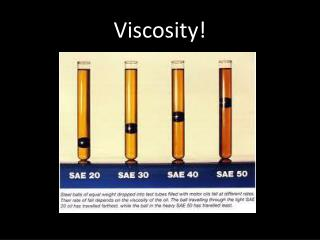DownloadDownload PresentationViscosity!

# Viscosity!

Download Presentation## Viscosity!

- - - - - - - - - - - - - - - - - - - - - - - - - - - E N D - - - - - - - - - - - - - - - - - - - - - - - - - - -
##### Presentation Transcript

1. Viscosity!

2. Viscosity (粘性) is how THICK or THIN a fluid is.

3. Flow rate (流量) is how fast the liquid flows (流).

4. We also need to understand molecules. Molecules are like particles that are stuck together.

5. If flow rate is slow, viscosity is _____. high So the molecules are _______. bigger If the flow rate is fast, viscosity is____. low So the molecules are _______. smaller

6. Viscosity changes when the liquid is cold or hot.

7. Variables! The Constant Variable: WE DON’T CHANGE! WE MUST NOT CHANGE!The Independent Variable: WE CHANGE! WE MUST CHANGE!The Dependent Variable: THIS IS OUR ANSWER!

8. Balanced Forces: Force that is equal and opposite. (The same and coming from both sides). Unbalanced Forces: Force that is unequal and opposite. (A strong force on one side, a weak force on the other.) No the particles did not move closer together, just the air came out.

9. Mr. Wu’s mass is 50 on the moon. kg Mr. Wu’s weight is 49 on the Moon. newtons P = F/A So, the force is 12,00 newtons And the area is 42.5 so the pressure is… 28.2 pascals

10. 1. Gravity2. Friction3. Tension The force is balanced if the boy cannot lift the bag. If he can lift the bag, it is unbalanced.

11. Adhesion!

12. Some molecules are positive, some are negative.

13. Cohesion

14. So…

15. Watch this!

16. Is the paper clip denser than the water? Yes, it is!

17. Why did the paper clip float on the water? Surface Tension! There is cohesion between the water particles that holds it together.

18. Surface Tension

19. How did the paper clip float on water?

20. Let’s play a game! Adhesion or cohesion!? Cohesion - the bubble’s particles stick to each other.

21. Adhesion or cohesion!? Adhesion – the water particles stick to the glass particles

22. This glue bubble? Cohesion – the glue particles stick to each other

23. Glue the paper to the wall? Adhesion - the glue sticks to the wall

24. Think of an example of either cohesion or adhesion.

25. Explosion!

26. Implosion! Implode!

27. Why did the can implode? What happens to particles when they lose energy (cool down)? What about the air particles? Are they moving faster or slower?

28. Balanced Forces: Force that is equal and opposite. (The same and coming from both sides). Unbalanced Forces: Force that is unequal and opposite. (A strong force on one side, a weak force on the other.) No the particles did not move closer together, just the air came out.

29. Mr. Wu’s mass is 50 on the moon. kg Mr. Wu’s weight is 49 on the Moon. newtons P = F/A So, the force is 12,00 newtons And the area is 42.5 so the pressure is… 28.2 pascals

30. 1. Matter • Solid, liquid, gas, viscosity, • Cohesion, adhesion, 2. Forces • friction, elastic force, static electricity • tension, magnetic force, gravity, balanced • and unbalanced, compression, deformation, • pressure

31. Fluids (流体) – liquids and gases.

32. But liquids and gases… We can make them work for us…

33. Fluids Under Pressure (压力) Under pressure!

34. Fluids flow from high pressure areas to low pressure areas.

35. How does a juice box (果汁盒) work?

36. Area of low pressure.

37. Area of higher pressure.

38. So the juice goes from high pressure to low pressure.

39. So, what about the forces? Balanced? Unbalanced!

40. If you climb a mountain very quickly, this might happen:

41. Why?

42. The air pressure inside your head is the same but outside it is much less. Probably, just your ears will “pop”

43. What if there is no pressure? Vacuum - 真空 – an area with very low pressure. A perfect vacuum has no pressure.

44. How does it work The room pressure is the same so it is higher, the air goes in the machine. The machine pushes out the air making a low pressure area.

45. If you go deep underwater, the pressure is more.

46. If you go deep underwater, the pressure is more.

47. Remember me? 帅哥？ Pascals are small so we need KILOPASCALS!